# a If there are 6 people in a room, find the probability at least 2 were...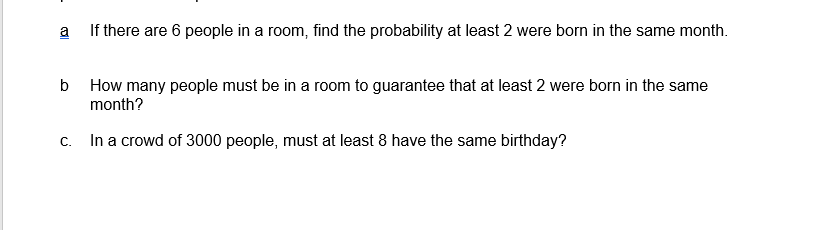a If there are 6 people in a room, find the probability at least 2 were born in the same month. b How many people must be in a room to guarantee that at least 2 were born in the same month? C. In a crowd of 3000 people, must at least 8 have the same birthday?

This Homework Help Question: "a If there are 6 people in a room, find the probability at least 2 were..." No answers yet.

We need 10 more requests to produce the answer to this homework help question. Share with your friends to get the answer faster!

0 /10 have requested the answer to this homework help question.

Once 10 people have made a request, the answer to this question will be available in 1-2 days.
All students who have requested the answer will be notified once they are available.
##### Add Answer to: a If there are 6 people in a room, find the probability at least 2 were...
Similar Homework Help Questions
• ### What is the probability that in a room of n people, at least three of them...

What is the probability that in a room of n people, at least three of them have the same birthday? Explain all the terms in your solution. What is the fewest amount of people such that the probability of at least three of these people having the same birthday is greater than 1/3? You will have to code your solution from the first question and plug in values for n. Include the code snipped you used to solve this.

• ### 2) There are n persons in a room. (a) What is the probability that at least...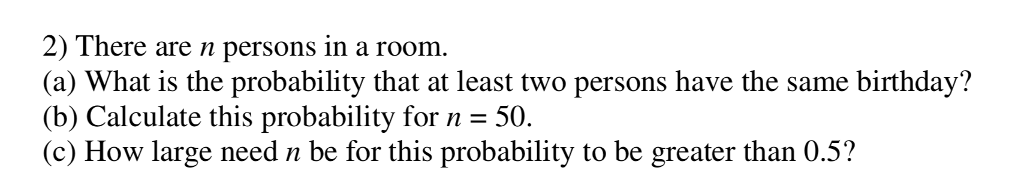2) There are n persons in a room. (a) What is the probability that at least two persons have the same birthday? (b) Calculate this probability for n 50. (c) How large need n be for this probability to be greater than 0.5?

• ### please help me understand this problem. k people in a room. We would like to find...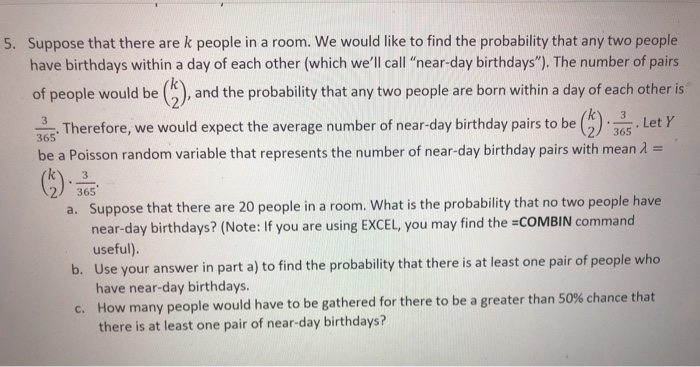please help me understand this problem. k people in a room. We would like to find the probability that any two people 5. Suppose that there are have birthdays within a day of each other (which we'll call "near-day birthdays"). The number of pairs and the probability that any two people are born within a day of each other is of people would be S4Therefore, we would expect the average number of near-day birthday pairs to be be a Poisson...

• ### The answer is 0.7063 32. What is the probability that at least two people in a...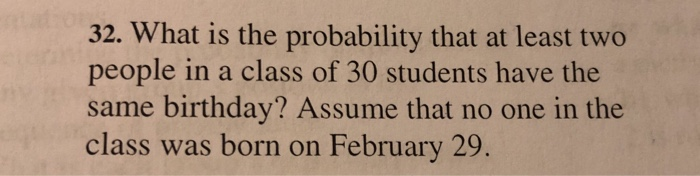The answer is 0.7063 32. What is the probability that at least two people in a class of 30 students have the same birthday? Assume that no one in the class was born on February 29.

• ### We proved in class that in a group of 23 people, the probability of two people...

We proved in class that in a group of 23 people, the probability of two people having the same birthday is 0.5073. Also, in a group of 100 people, the probability of two people having the same birthday is 0.9999998.   On late-night television’s The Tonight Show with Johnny Carson (on air during 1962-1992), Carson was discussing the birthday problem. At a certain point, he remarked to his audience of approximately 100 people “Great! There must be someone here who was...

• ### Let ?? be the probability that in a group of ? people, at least two share...

Let ?? be the probability that in a group of ? people, at least two share the same birthday. Assume there are 365 days in a year, and that all birthdays are equally likely. a) What is the probability that in a group of 2, 3, 4, or 5 people, at least two have the same birthday? ?2=? ?3=? ?4=? ?5=?

• ### The R command sample (1:365,23, replace=T) > simulates birthdays from a group of 23 people. The...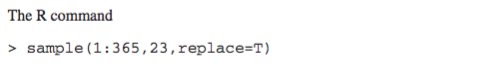The R command sample (1:365,23, replace=T) > simulates birthdays from a group of 23 people. The expression 2 %int table (sample (1:365, 23, replace=T)) can be used to simulate the birthday problem. It creates a frequency table showing how many people have each birthday, and then determines if two is in that table; that is, whether two or more people have the same birthday. Use and suitably modify the expression for the following problems (a) Simulate the probability that two...

• ### 5.36. (a) In a group of 23 strangers, what is the probability that at least two of bout if there ...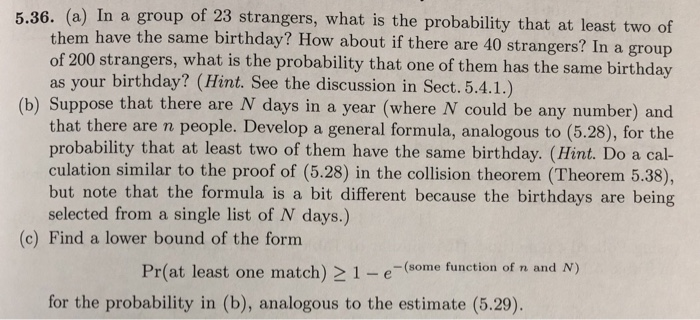5.36. (a) In a group of 23 strangers, what is the probability that at least two of bout if there are 40 strangers? In a group them have the same birthday? How a of 200 strangers, what is the probability that one of them has the same birthday as your birthday? (Hint. See the discussion in Sect. 5.4.1.) (b) Suppose that there are N days in a year (where N could be any number) and that there are n people....

• ### Find the probability of at least one birthday match among a group of 48 people

Find the probability of at least one birthday match among a group of 48 people.

• ### I need the answer a, b, c. you have to annwer part C must a) A...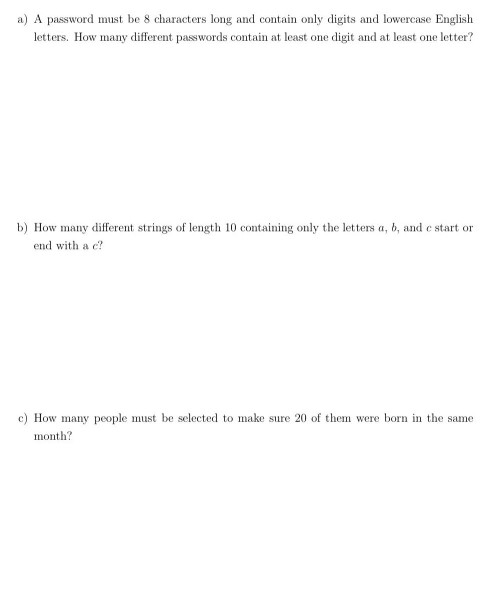I need the answer a, b, c. you have to annwer part C must a) A password must be 8 characters long and contain only digits and lowercase English letters. How many different passwords contain at least one digit and at least one letter? b) How many different strings of length 10 containing only the letters a, b, and c start or end with a c? c) How many people must be selected to make sure 20 of them were...

Free Homework App